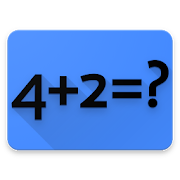Math ExercisesEveryone
81
·
Offers in-app purchases
Do you have difficulties with maths? This app will help you. It has been designed to assist you in training your maths skills.

Maths problem types
➖ Subtraction - minus
✖️ Multiplication - times
➗ Division - divide by
➗ Division with remainders
+ others

This maths app includes courses for various mathematical problems, as shown below:

Subtraction
Subtracting from 10
Subtracting from 20
Subtracting from 100
Subtracting from 1000

Multiplication levels
Multiplying with numbers up to 10
Multiplying with numbers up to 12
Multiplying with numbers up to 20

Division levels
Division with numbers up to 10
Division with numbers up to 12
Division with numbers up to 20

Multiplication tables
Tables up to 10
Tables over 10

Maths - how to use
This app will guide you through maths examples in a simple way, train your calculus skills, and help you improve quickly. It shows you four results from which you have to choose the right one.

Thanks to this maths app you won’t need any private lessons anymore. Choose your own learning schedule. From now on you can quit using a calculator for mental calculations.

It may surprise you but your children probably have better mental calculation skills than you. You could try to compare your fundamental calculation skills with them.

Apparently, division with remainder is an area where mistakes are often made.

We need these basic mathematical and arithmetic operations in our everyday life. For example, when calculating loans, instalments, discounts and pocket money for children. Without this fundamental knowledge in maths, it is almost impossible to get a good job.

This maths application works on the principle of multiple choice flash cards. You can choose from different types of maths problems and select the complexity correspondingly. An example for this is the possibility to train addition up to ten in the first grade level (addition up to 10) then up to 20 in the second grade level, up to 100 in the third grade level and even up to 1000 in the fourth grade level. During each exercise four cards will be revealed – one of them holds the correct answer. Your task is to choose the right one each time.

For each correct answer you will receive points that add up to a final score (points). According to the points, you will move up or down in levels.

This levelling system was deliberately added to give the game a certain incentive element.
Collapse

Review Policy
4.0
81 total
5
4
3
2
1

What's New

new game, Column subtraction
Collapse

Updated
May 29, 2019
Size
4.6M
Installs
50,000+
Current Version
6.5.5
Requires Android
4.2 and up
Content Rating
Everyone
In-app Products
\$0.99 per item
Permissions
Offered By
Zdenek Jonas
Developer
Pod Hájem 322 Králův Dvůr 267 01 Česká republika